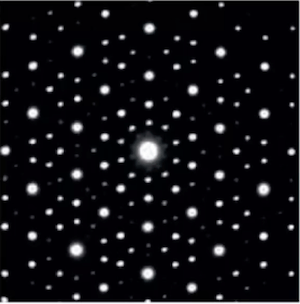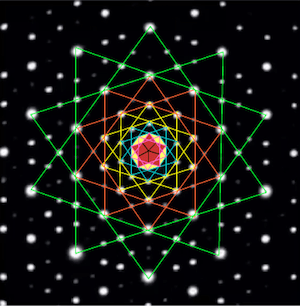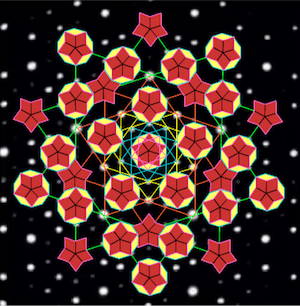# This month’s topics:

### Quasi-crystalline tilingsDetail of the ceiling of the pavilion at the Tomb of Hafez in Shiraz, Iran. (Full image here). Note the 10-fold local rotational symmetry: a periodic or “crystalline” tiling cannot have a global symmetry of that order. Image courtesy of Stephanie Hawkins.

On April 6, 2021 the Museum of Nature & Science in Las Cruces, NM presented a Zoom lecture by Rima Ajlouni (School of Architecture, University of Utah) with the title “Quasi-crystalline Geometry in Islamic Art and Architecture.” (The lecture is now available on Facebook).

This presentation is an attempt to reverse-engineer the remarkable non-periodic tilings found in medieval islamic monuments: how they were constructed with the the tools available at the time. In the process Prof. Ajlouni eleganty exhibits the connection between Penrose tilings and quasi-crystals, starting from the original April 8, 1982 electron diffraction scan that ultimately won Daniel Shechtman his Nobel prize:Electron diffraction scan from a sample of an aluminum-manganese alloy. Original source: Phys. Rev. Lett. 53, 1951-1953 (1982); from a still in Prof. Ajlouni’s video, used with permission.

The process starts by observing in Shechtman’s image a series of nested decagons, each of which (joining every third vertex) gives a 10-pointed star containing the next smaller one. In a series of five, the innermost partitions naturally into the union of ten Penrose tiles, here colored red and yellow. This union, together with the smaller group of five red tiles, forms the seeds of the tiling.Concentric 10-pointed stars superimposed on Shechtman’s scan; the innermost one with its outer decagon has been filled in with ten Penrose tiles.

In the next step, one or the other of the seeds is planted at a symmetrical subset (the hidden grid) of the vertices of the outer two stars.21 of the larger seeds and 10 of the smaller have been planted at points of the hidden grid.

The remaining open space is filled with symmetrically placed copies of the two connecting formations

yielding a Penrose tiling with 5-fold symmetry:Adding 45 copies of the yellow connecting formation, and 20 copies of the red, fills in all the open space and yields a Penrose tiling.

Prof. Ajlouni shows how this tiling, together with a star-shaped subset, can be used as seeds, with the same hidden grid and two analogously chosen filling configurations, to give a “larger” tiling (assuming the tile size to be constant) and that this hierarchical process can be continued to make arbitrarily large Penrose tilings.

### Fractal geometry of cities

“How the geometry of cities determines urban scaling laws,” by Carlos Molinero and Stefan Thurner, was published on March 18, 2021 in the Journal of the Royal Society Interface. They begin: “One of the surprising findings in urban science is that many of the hundreds of quantities and variables that characterize the dynamics, functioning, and performance of a city exhibit power law relations. These are called scaling laws, meaning that a quantity $X$ depends on a variable $p$ (such as population) in a power-law fashion. In particular, this means that $X$ is related to the population of the city as
$$X \propto p^{\gamma}$$
where $\gamma$ is the scaling exponent and $p$ represents population size.
“Scaling laws can often be explained directly from the geometry of the underlying structures of a system. Classic examples include Galileo’s understanding of the relation between the shape of animals and their body mass … or the scaling laws of river basins given by their fractal geometry. In the same spirit, we provide a simple and a direct geometrical explanation of urban scaling exponents, derived from the fractal geometry of cities.”

This study involves the concept of fractal dimension; one definition is the box dimension. Here is how the authors introduce it:“The length of the street network with fractal dimension $d_i$ expands with the linear scale $L$ as $\ell \propto L^{d_i}$.” Image from J. R. Soc. Interface. 18 20200705, used under CCA license

In this example from the article, the street grid of a city has fractional dimension $d_i$ if its length $\ell$ is proportional to $L^{d_i}$, where $L$ is the linear size of the city (so, for example, the city area varies as $L^2$).

“Cities across countries, latitudes and cultures are different—and so is their geometry. How should cities that are significantly different in their geometry lead to similar scaling exponents? To answer this question we focus on the ratio of two geometric aspects of a city, the fractal dimension [$d_i$] of its infrastructure (street networks), and the fractal dimension [$d_p$] of the population … . The fractal of the population can be imagined as the cloud of people that is obtained by identifying the position of every person in three dimensions.” One of the results reported is that even though those two fractal dimensions depend on the size of the population, their ratio stabilizes as population grows.“Fractal dimensions of the street network, $d_i$ (orange) and for the population, $d_p$ (blue) for 1000 cities in the UK as a function of their population size $p$. While the fractal dimensions are strongly size dependent, their ratio, $\gamma_{\rm sub} = d_i/d_p$ (black), is not. It is found to be approximately constant, $\gamma_{\rm sub}\sim 0.86$.” Image from J. R. Soc. Interface. 18 20200705, used under CCA license.

In a related result, the authors explain earlier measurements from other sources: “scaling laws with respect to population size were found for GDP, the number of patents, walking speed or crime rates. The associated scaling exponent for these relations appears to be in a range of $\gamma \sim 1.1 – 1.2$ … ,” by arguing that for these parameters, $\gamma$ should be equal to $2-\gamma_{\rm sub}$.